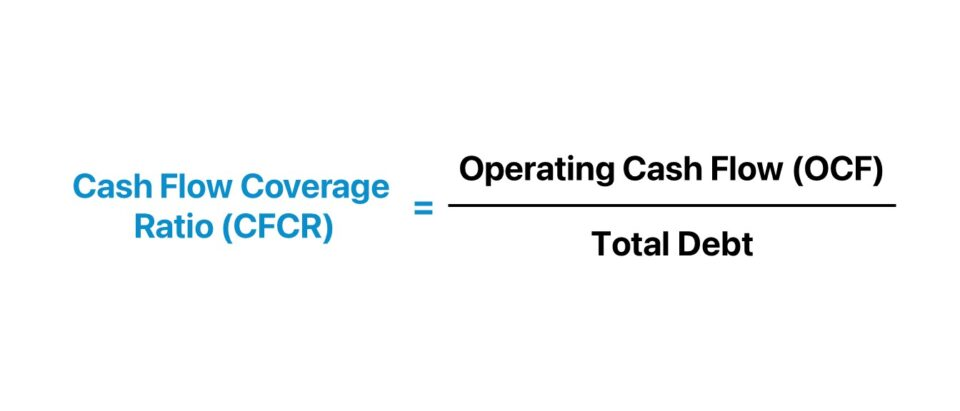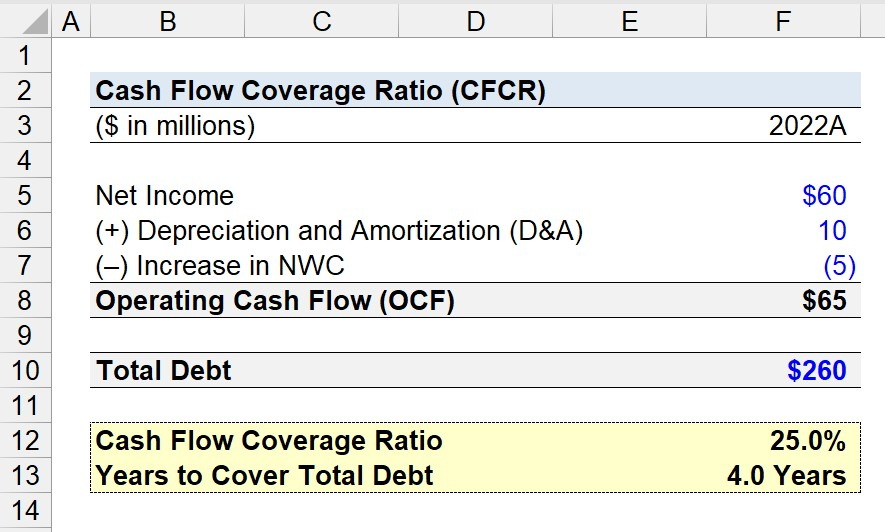Welcome to Wall Street Prep! Use code at checkout for 15% off.# Cash Flow Coverage Ratio

Guide to Understanding the Cash Flow Coverage Ratio (CFCR)## How to Calculate Cash Flow Coverage Ratio?

The cash flow coverage ratio (CFCR) measures the credit risk of a company by comparing its operating cash flow (OCF) to its total debt outstanding.

The CFCR reflects the relationship between a company’s operating cash flow (OCF) and total debt burden, which reflects its capacity to pay down its debt balance using its cash flows from operating activities.

The cash flow coverage ratio is calculated by dividing the operating cash flow (OCF) of a company by the total debt balance in the corresponding period.

From the perspective of evaluating the solvency of a borrower, a higher cash flow coverage ratio is preferable.

• Higher Cash Flow Coverage Ratio (CFCR) → Low Credit Risk and Less Risk of Default
• Lower Cash Flow Coverage Ratio (CFCR) → Higher Credit Risk and and Greater Risk of Default

Given the cash flow coverage ratio (CFCR), the number of years needed for the borrower to cover its entire debt obligation can be estimated, assuming its current level of operating cash flow (OCF) generation is sustained.

## Cash Flow Coverage Ratio Formula (CFCR)

The formula to calculate the cash flow coverage ratio (CFCR) is as follows.

Cash Flow Coverage Ratio (CFCR) = Operating Cash Flow (OCF) ÷ Total Debt

Where:

The estimated number of years required for the company to pay off its total debt balance is calculated by dividing one by the CFCR.

Years to Cover Total Debt = 1 ÷ Cash Flow Coverage Ratio (CFCR)

## Cash Flow Coverage Ratio Calculator

We’ll now move to a modeling exercise, which you can access by filling out the form below.Submitting...

## Cash Flow Coverage Ratio Calculation Example (CFCR)

Suppose we’re tasked with calculating the cash flow coverage ratio of a company to assess its current credit risk.

In 2022, the company generated \$60 million in net income and incurred \$10 million in depreciation and amortization (D&A), while its net working capital (NWC) increased by \$5 million.

• Net Income = \$60 million
• Depreciation and Amortization (D&A) = \$10 million
• Increase in NWC = (\$5 million)

The D&A expense is a non-cash item added back to net income, since there was no actual cash outflow.

Because an increase in net working capital (NWC) is an outflow of cash, the \$5 million increase is a begative adjustment to net income.

Given those figures, our company’s operating cash flow (OCF) is \$65 million.

• Operating Cash Flow (OCF) = \$60 million + \$10 million – \$5 million = \$65 million

If the total debt balance is assumed to be \$260 million, the cash flow coverage ratio is 25.0%.

• Total Debt = \$260 million
• Cash Flow Coverage Ratio (CFCR) = \$65 million ÷ \$260 million = 25.0%

The 25.0% CFCR means the operating cash flow (OCF) of our company can cover a quarter of the total debt balance.

After dividing one by our company’s cash flow coverage ratio (CFCR), the time necessary for the company’s operating cash flow (OCF) to fulfill its total debt balance is implied to be 4 years.

• Years to Cover Total Debt = 1 ÷ 25.0% = 4.0 YearsStep-by-Step Online Course

### Everything You Need To Master Financial Modeling

Enroll in The Premium Package: Learn Financial Statement Modeling, DCF, M&A, LBO and Comps. The same training program used at top investment banks.